2017/01/07 19:33

# MNIST机器学习入门

MNIST是一个入门级的计算机视觉数据集，它包含各种手写数字图片：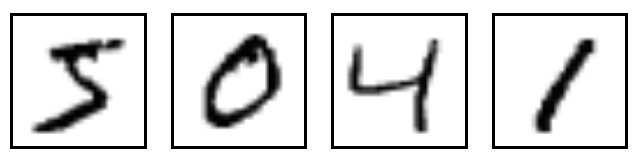## MNIST数据集

MNIST数据集的官网是Yann LeCun's website。在这里，我们提供了一份python源代码用于自动下载和安装这个数据集。你可以下载这份代码，然后用下面的代码导入到你的项目里面，也可以直接复制粘贴到你的代码文件里面。

``````import tensorflow.examples.tutorials.mnist.input_data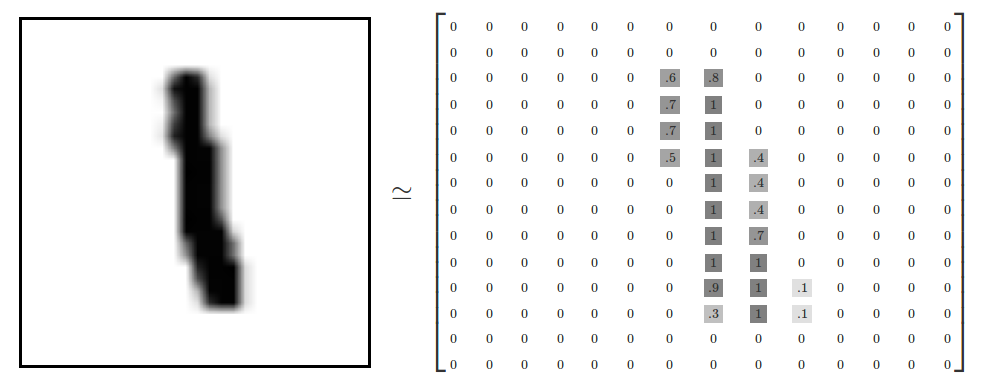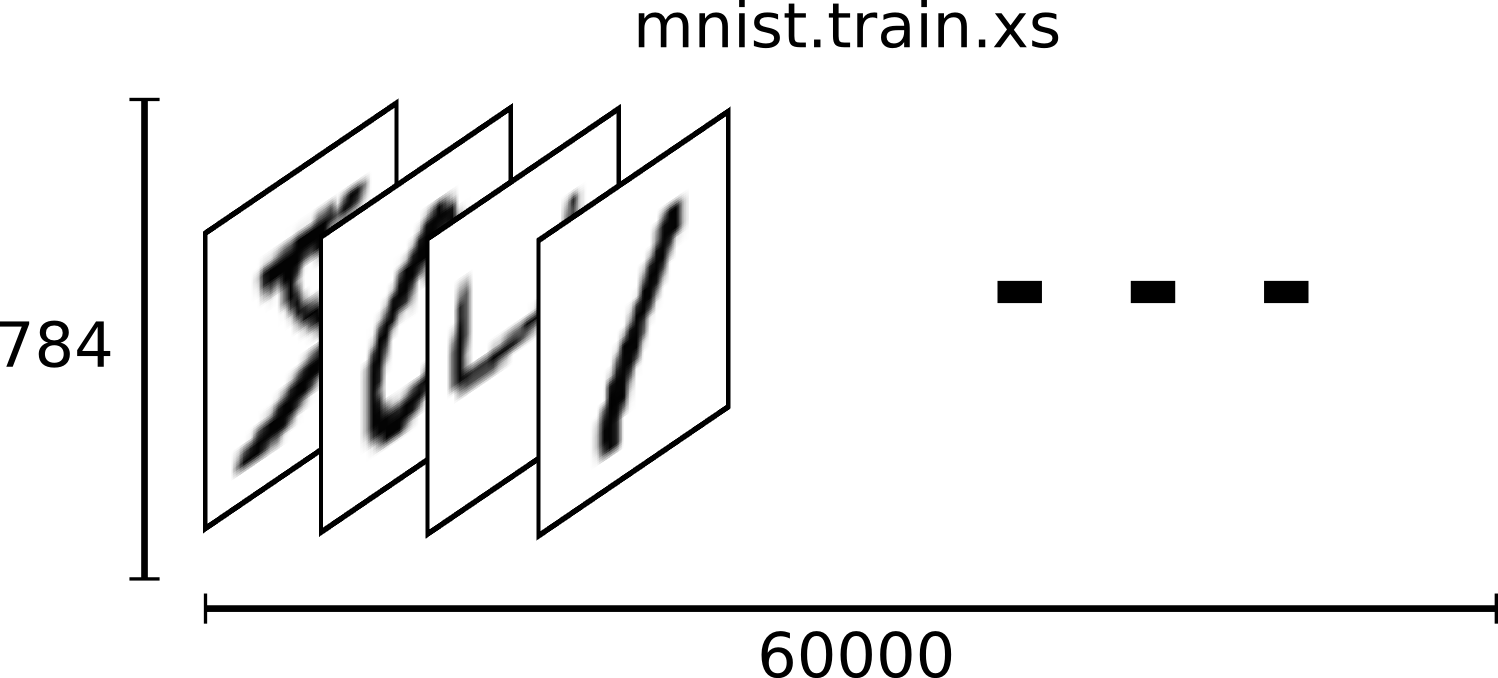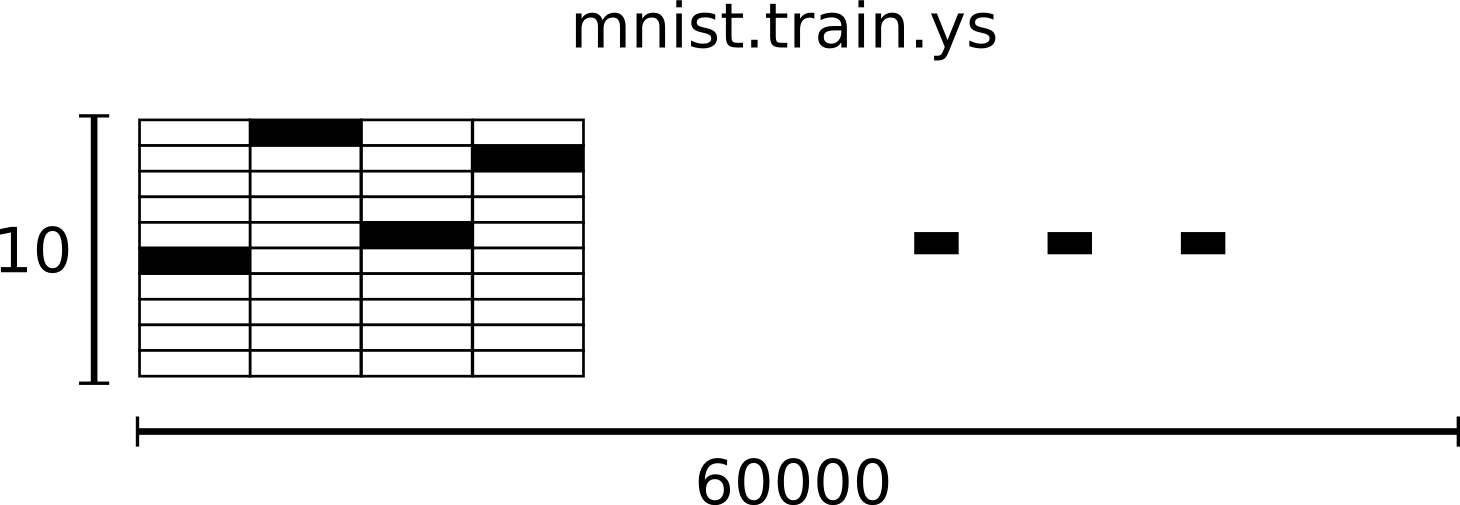## Softmax回归介绍

softmax回归（softmax regression）分两步：第一步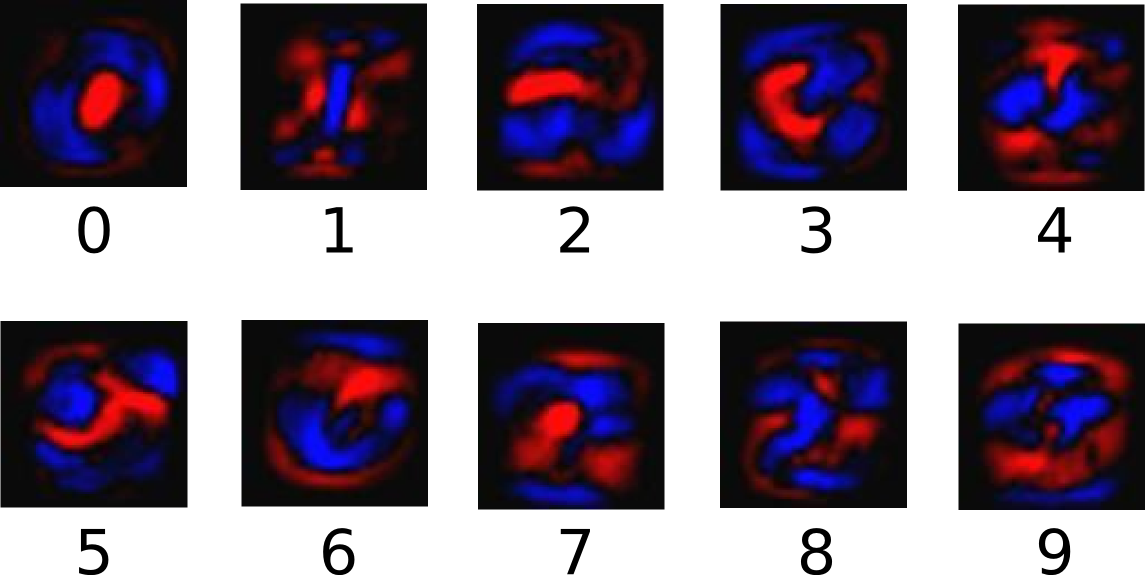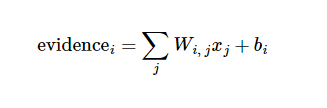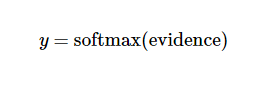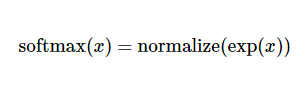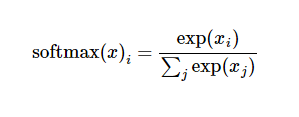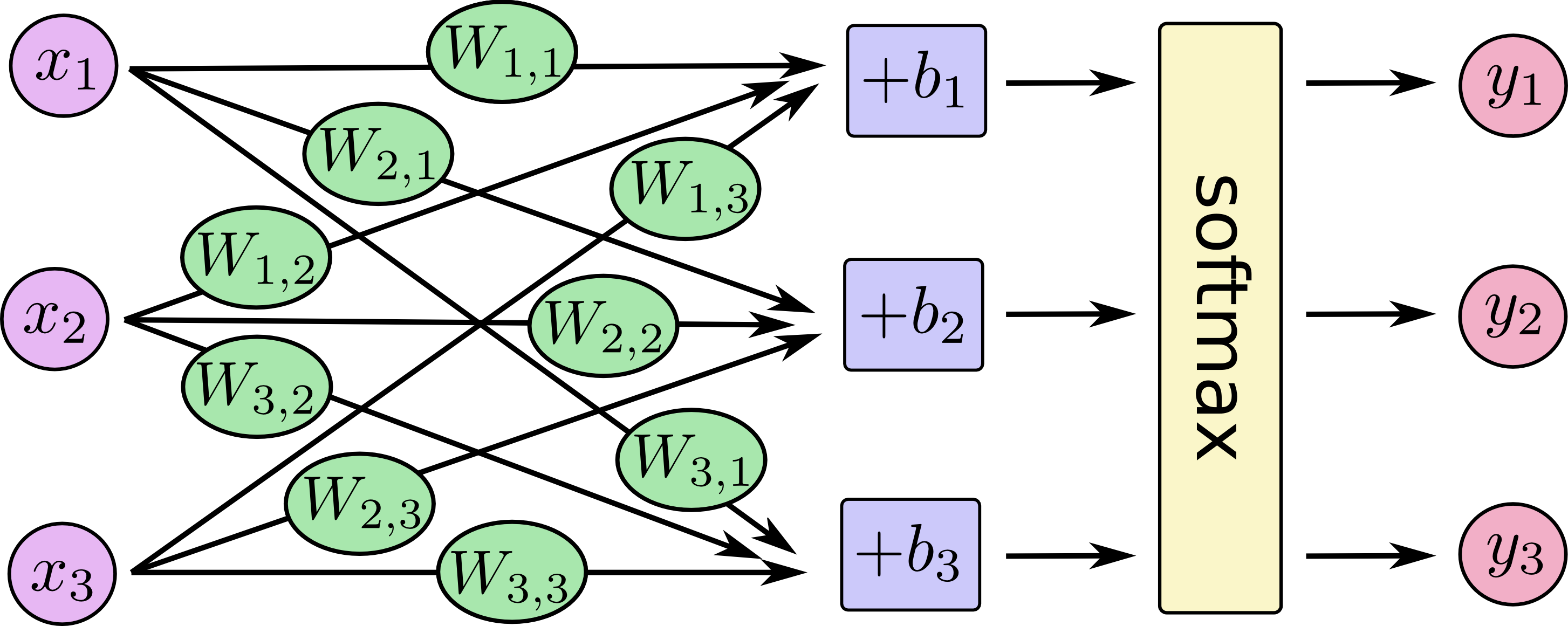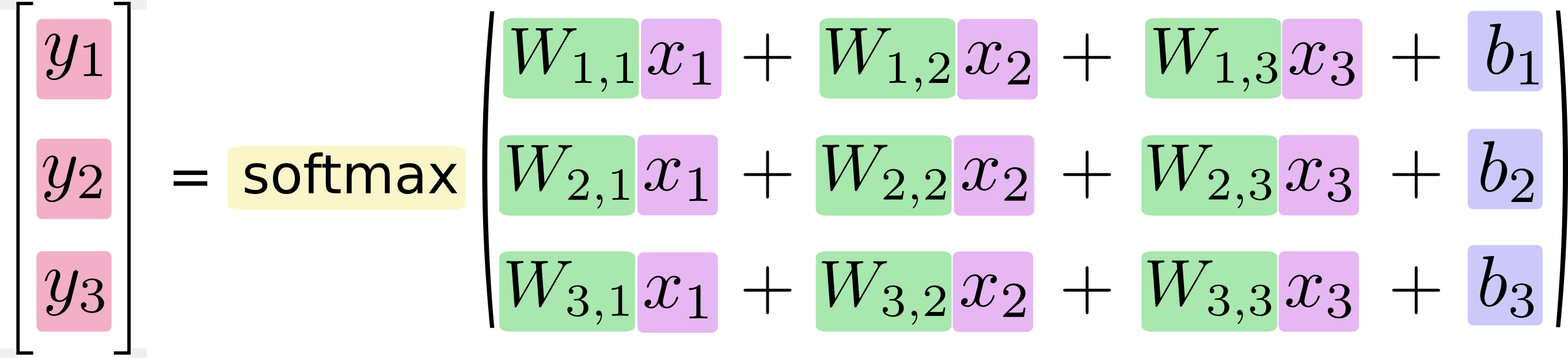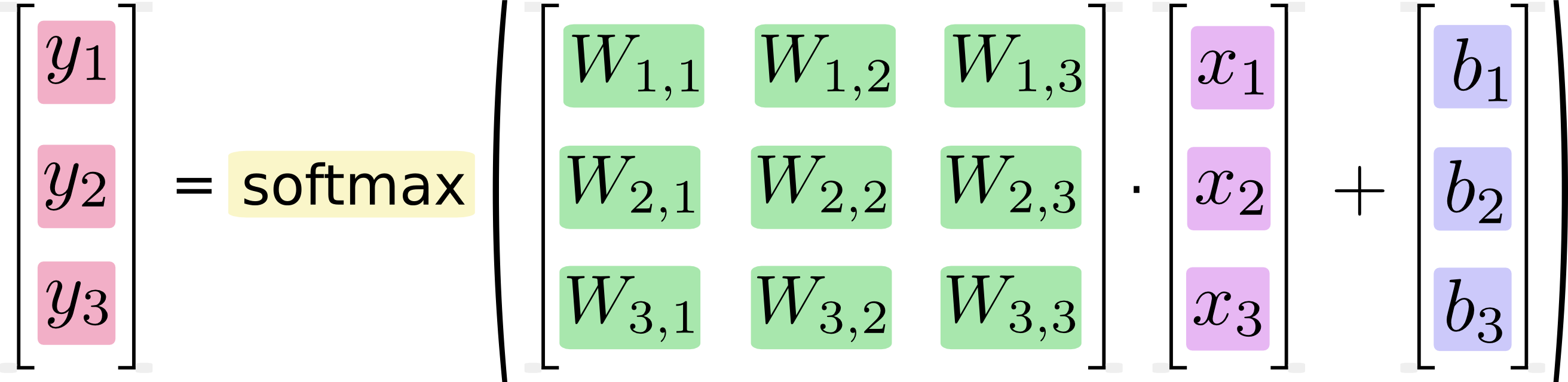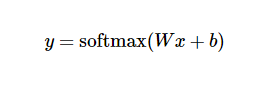## 实现回归模型

TensorFlow也把复杂的计算放在python之外完成，但是为了避免前面说的那些开销，它做了进一步完善。Tensorflow不单独地运行单一的复杂计算，而是让我们可以先用图描述一系列可交互的计算操作，然后全部一起在Python之外运行。（这样类似的运行方式，可以在不少的机器学习库中看到。）

``import tensorflow as tf``

``x = tf.placeholder(tf.float32, [None, 784])``

`x`不是一个特定的值，而是一个占位符`placeholder`，我们在TensorFlow运行计算时输入这个值。我们希望能够输入任意数量的MNIST图像，每一张图展平成784维的向量。我们用2维的浮点数张量来表示这些图，这个张量的形状是`[None，784 ]`。（这里的`None`表示此张量的第一个维度可以是任何长度的。）

``````W = tf.Variable(tf.zeros([784,10]))
b = tf.Variable(tf.zeros())``````

``y = tf.nn.softmax(tf.matmul(x,W) + b)``

## 训练模型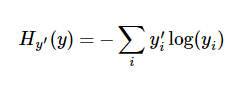y 是我们预测的概率分布, y' 是实际的分布（我们输入的one-hot vector)。比较粗糙的理解是，交叉熵是用来衡量我们的预测用于描述真相的低效性。更详细的关于交叉熵的解释超出本教程的范畴，但是你很有必要好好理解它

``y_ = tf.placeholder("float", [None,10])``

``cross_entropy = -tf.reduce_sum(y_*tf.log(y))``

``train_step = tf.train.GradientDescentOptimizer(0.01).minimize(cross_entropy)``

TensorFlow在这里实际上所做的是，它会在后台给描述你的计算的那张图里面增加一系列新的计算操作单元用于实现反向传播算法和梯度下降算法。然后，它返回给你的只是一个单一的操作，当运行这个操作时，它用梯度下降算法训练你的模型，微调你的变量，不断减少成本。

``init = tf.initialize_all_variables()``

``````sess = tf.Session()
sess.run(init)``````

``````for i in range(1000):
batch_xs, batch_ys = mnist.train.next_batch(100)
sess.run(train_step, feed_dict={x: batch_xs, y_: batch_ys})``````

## 评估我们的模型

``correct_prediction = tf.equal(tf.argmax(y,1), tf.argmax(y_,1))``

``accuracy = tf.reduce_mean(tf.cast(correct_prediction, "float"))``

``print sess.run(accuracy, feed_dict={x: mnist.test.images, y_: mnist.test.labels})``

0
1 收藏

### 作者的其它热门文章0 评论
1 收藏
0